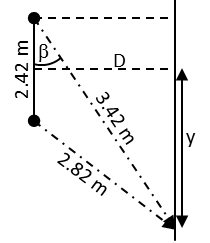# Two loudspeakers are 2.42 m apart. A person stands 3.42 m from one speaker and 2.82 m from the...

## Question:

Two loudspeakers are 2.42 m apart. A person stands 3.42 m from one speaker and 2.82 m from the other.

What is the lowest frequency at which destructive interference (sound cancellation) will occur at this point?

## Sound interference

This problem is the equivalent of Young's double slit experiment, only with sound waves. In this case it is necessary to use the interference minimum equation and keep in mind that the wavelength is equal to the speed of propagation of sound in the air divided by the frequency of the wave.

{eq}d\sin\theta=\left (m+\frac{1}{2} \right )\lambda=\left (m+\frac{1}{2} \right )\frac{v}{f} {/eq}

First we will calculate the distance that separates the person from the center of the interference pattern and then the distance that separates the speakers from the plane where the interference pattern is being heard.{eq}\mathrm{(2.82\,m)^2=(2.42\,m)^2+(3.42\,m)^2-2(2.42\,m)(3.42\,m)cos\beta\Rightarrow \beta=arccos\left ( \frac{(2.42\,m)^2+(3.42\,m)^2-(2.82\,m)^2}{2(2.42\,m)(3.42\,m)} \right )=54.5^\circ}\\ \sin(90.0^\circ-54.5^\circ)=\frac{y+(2.42\,\mathrm{m})/2}{3.42\,\mathrm{m}}\Rightarrow y=\mathrm{(3.42\,m)sin(90.0^\circ-54.5^\circ)-\frac{2.42\,m}{2}=0.78\,m}\\ \cos(90.0^\circ-54.5^\circ)=\frac{D}{3.42\,\mathrm{m}}\Rightarrow D=\mathrm{(3.42\,m)cos(90.0^\circ-54.5^\circ)=2.78\,m} {/eq}

Using now the equation corresponding to the interference minimums, we can find the minimum frequency at which destructive interference occurs:

{eq}\left.\begin{matrix} d\sin\theta=\left (m+\frac{1}{2} \right )\frac{v}{f}\\ \sin\theta=\frac{1}{\sqrt{(D/y)^2+1}}\\ m=0 \end{matrix}\right\}\Rightarrow f=\frac{v}{2d}\sqrt{\left ( \frac{D}{y} \right )^2+1}\\ \therefore f=\mathrm{\frac{343\,m/s}{2(2.42\,m)}\sqrt{\left ( \frac{2.78\,m}{0.78\,m} \right )^2+1}=262\,Hz} {/eq}